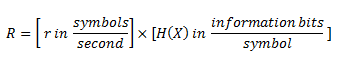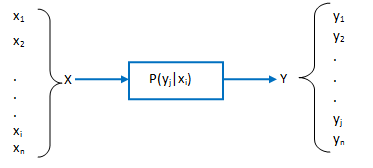# Interview Questions and Answers on Information Theory

### Q.1. What is the meaning of word ‘Information ‘ ?

Ans. The amount of information received from the knowledge of occurrence of a event may be related to the likelihood or probability of occurrence of that event. The message associated with the least likelihood event thus consists of maximum information. The above amount of information in a message depends only upon the uncertainty of the underlying event rather than its actual content .

### Q.2. What are Information Soruce ? Explain .

Ans. An information source may be viewed as an object which produces an event, the outcome of which is selected at random according to a probability distribution.

A practical source in a communication system is a device which produces messages, and it can either analog or discrete. Here, we deal mainly with the discrete sources since analog sources can be transformed to discrete sources through the use of sampling and quantization techniques.

As a matter of fact, a discrete information source is a source which has only a finite set of symbols as possible outputs. The set of source symbols is called the source alphabet and the elements of the set are called symbols or letters.

Information sources can be classified as having memory or being memoryless. A source with memory is one for which a current symbol depends on the previous symbols. A memoryless source is one for which each symbol produced is independent of the previous symbols.

A discrete memoryless source (DMS) can be characterized by the list of the symbols, the probability assigned to these symbols, and the specification of the rate of generating these symbols by the source .

### Q.3. Define the information content of a symbol.

Ans. The information content of a symbol xi, denoted by I(xi) is defined byWhere P(xi) is the probability of occurrence of symbol x.

### Q.4. What do you mean by Information rate ? Explain .

Ans. If the time rate at which source X emits symbols is r (symbols s), the information rate R of the source is given by

R = r H(X)  Bits/sec

Here R is information rate

H(X) is Entropy or average information

and r is rate at which symbols are generated.

Information rate R is represented in average number of bits of information per second. It is calculated as under :R = Information bits/ second

### Q.5. What is a Discrete Memoryless Channel (DMC) ? Explain .

Ans. A communication channel may be defined as the path or medium through which the symbols flow to the receiver end. A discrete memoryless channel (DMC) is a statistical model with an input X and an output Y as shown in figure.1 .During each unit of the time (signaling interval), the channel accepts an input symbol from X, and in response it generated an output symbol from Y. The channel is said to be discrete when the alphabets of X and Y are both finite. Also, it is said to be memoryless when the current output depends only on the current input and not on any of the previous inputs.